# Appendix B. Closed Form of a Geometric Series

In the literature of digital signal processing, we often encounter geometric series expressions like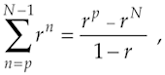or

Equation B-2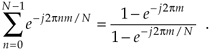Unfortunately, many authors make a statement like "and we know that," and drop Eqs. (B-1) or (B-2) on the unsuspecting reader who's expected to accept these expressions on faith. Assuming that you don't have a Ph.D. in mathematics, you may wonder exactly what arithmetic sleight of hand allows us to arrive at Eqs. (B-1) or (B-2). To answer this question, let's consider a general expression for a geometric series such as

Equation B-3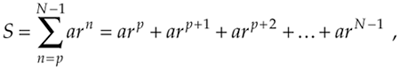where n, N, and p are integers and a and r are any constants. Multiplying Eq. (B-3) by r, gives us

Equation B-4Subtracting Eq. (B-4) from Eq. (B-3) gives the expression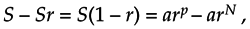or

Equation B-5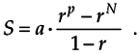So here's what we're after. The closed form of the series is

Equation B-6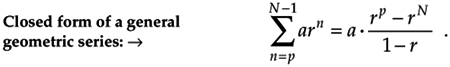(By closed form, we mean taking an infinite series and converting it to a simpler mathematical form without the summation.) When a = 1, Eq. (B-6) validates Eq. (B-1). We can quickly verify Eq. (B-6) with an example. Letting N = 5, p = 0, a = 2, and r = 3, for example, we can create the following list:

n

arn = 2 · 3n

0

2 · 30 = 2

1

2 · 31 = 6

2

2 · 32 = 18

3

2 · 33 = 54

4

2 · 34 = 162

The sum of this column is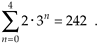Plugging our example N, p, a, and r values into Eq. (B-6),

Equation B-7which equals the sum of the rightmost column in the list above.

As a final step, the terms of our earlier Eq. (B-2) are in the form of Eq. (B-6) as p = 0, a = 1, and r = e–j2pm/N.[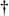] So plugging those terms from Eq. (B-2) into Eq. (B-6) gives us

[] From the math identity axy = (ax)y, we can say e–j2pnm/N = (e–j2pm/N)n, so r = e–j2pm/N.

Equation B-8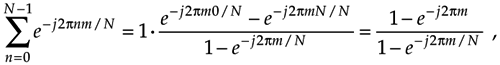confirming Eq. (B-2).

URL http://proquest.safaribooksonline.com/0131089897/app02Amazon